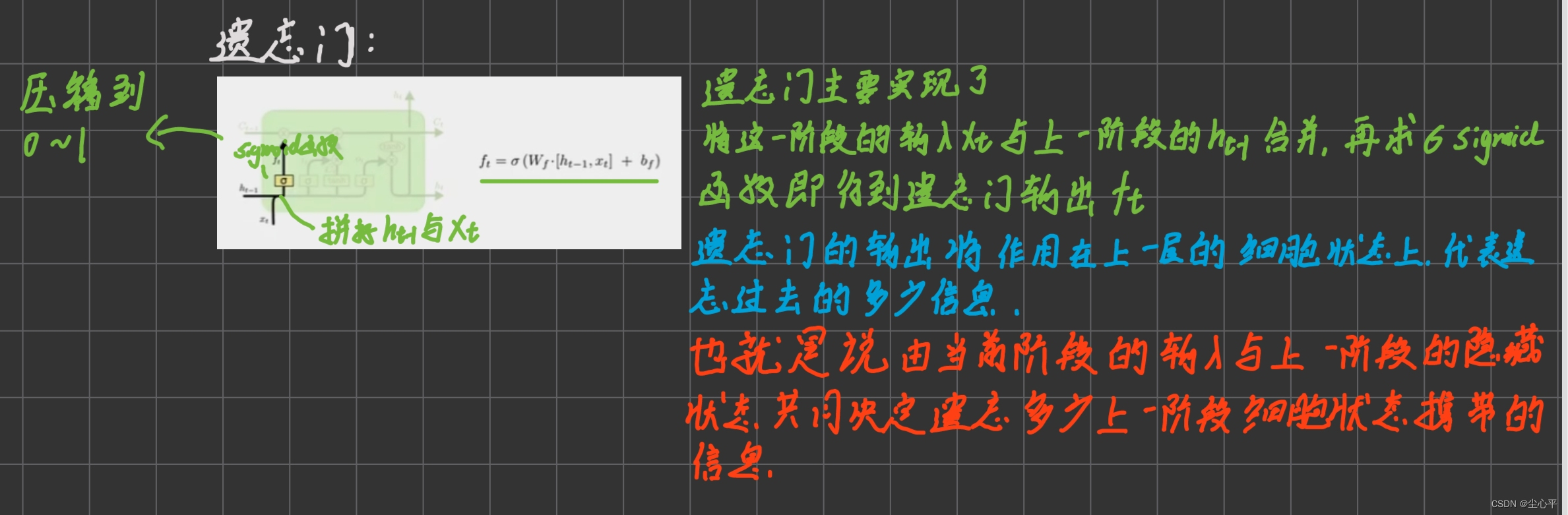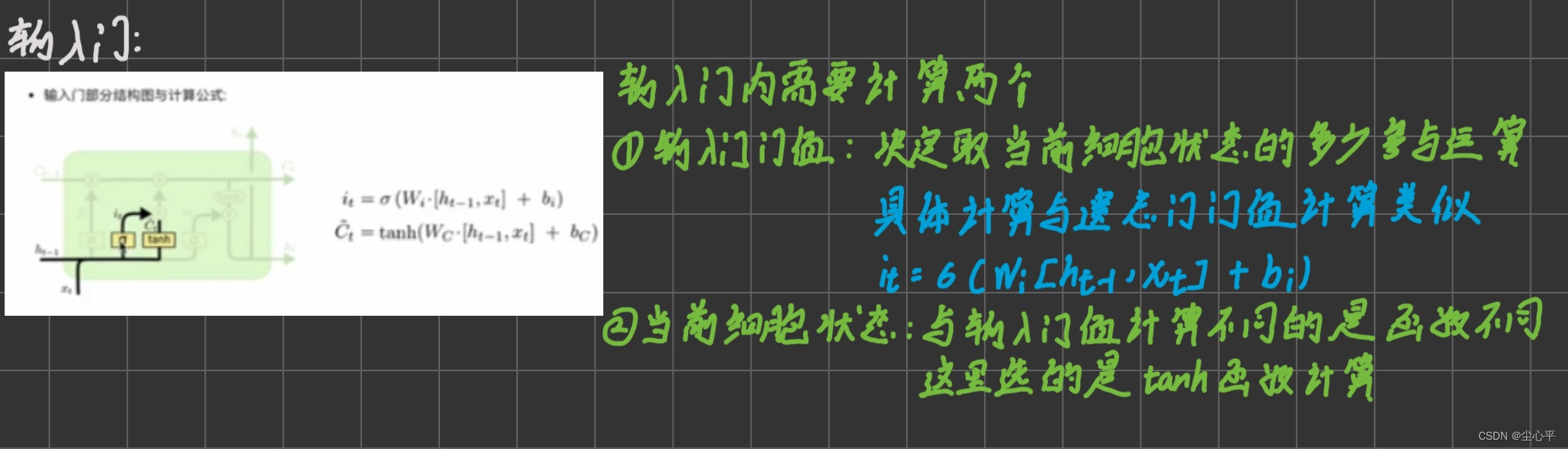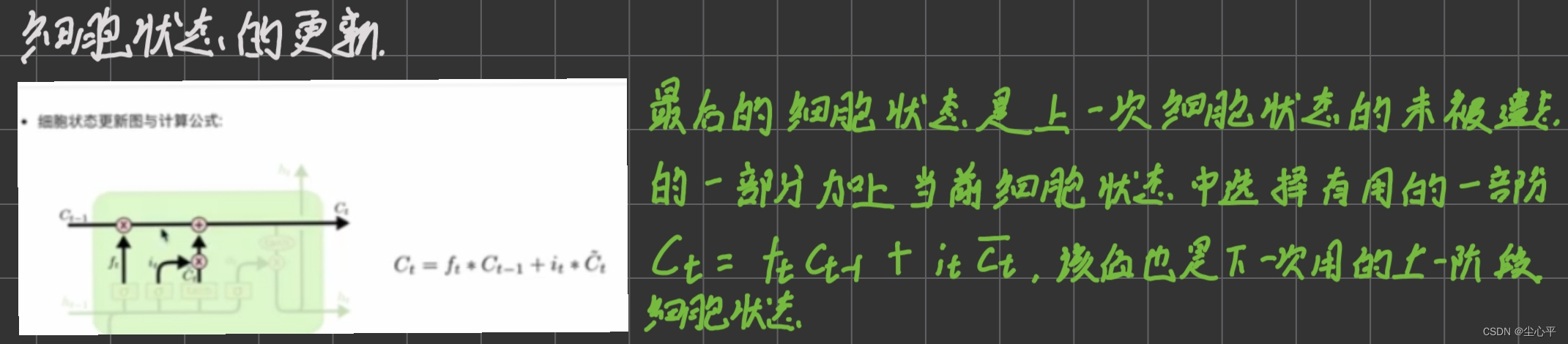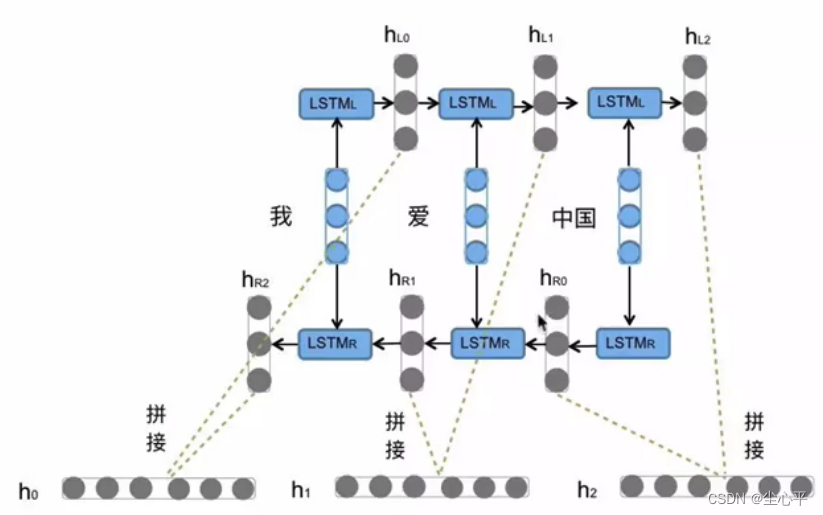# 深度学习——循环神经网络RNN

​活动地址：CSDN21天学习挑战赛

RNN网络结构

RNN的分类

# 什么是循环神经网络

## 为什么需要RNN

RNN的这种特点，使得它在解决语音识别、文本识别以及情感分析等NLP领域的问题时很有效果，也可以和CNN相结合解决一些连续性的CV问题

## RNN的分类

### 按输入输出的结构分类

• N输入—N输出

N输入即输入序列长度为N

N输出即每一次隐藏层处理后的输出层的输出均为最后输出结果的一部分

• N输入—1输出

N输入仍为网络模型每次递归的输入均为序列的不同部分，序列长n

1输出即只取网络模型最后一次递归的输出层的输出为整个模型的输出

• 1输入—N输出

1输入即网络模型每次递归共用同一个输入

N输出即每一次隐藏层处理后的输出层的输出均为最后输出结果的一部分

• N输入—M输出

N输入—M输出型网络模型是 N输入—1输出模型 与 1输入—N输出模型 的结合

### 传统RNN

​​​​​​​

tensorflow搭建传统RNN：

def __init__(self,
units,
activation='tanh',
use_bias=True,
kernel_initializer='glorot_uniform',
recurrent_initializer='orthogonal',
bias_initializer='zeros',
kernel_regularizer=None,
recurrent_regularizer=None,
bias_regularizer=None,
activity_regularizer=None,
kernel_constraint=None,
recurrent_constraint=None,
bias_constraint=None,
dropout=0.,
recurrent_dropout=0.,
return_sequences=False,
return_state=False,
go_backwards=False,
stateful=False,
unroll=False,
**kwargs):

units 正整数,输出空间的维度,一般为2的n次幂
activation 激活函数，默认值：双曲正切（ tanh ），功能为压缩值在－1~1范围内
use_bias bool型，默认值为 True ，表示使用偏置参数。
kernel_initializer 权重矩阵的初始化器，默认值是 glorot_uniform 。
recurrent_initializer 用于递归时权重矩阵的初始化器，默认值是 orthogonal 。
bias_initializer 偏置参数的初始化器。默认值是 zeros 。
kernel_regularizer  kernel 权重矩阵的正则化器，可以减小过拟合。默认值： None 。
recurrent_regularizer recurrent_kernel 权重矩阵的正则化器。默认值： None 。
bias_regularizer 偏置参数的正则化器。默认值： None 。
activity_regularizer 正则化功能应用于图层的输出（其“激活”）。默认值： None 。
kernel_constraint 约束函数应用于 kernel 权重矩阵。默认值： None 。
recurrent_constraint 约束函数应用于 recurrent_kernel 权重矩阵。默认值： None 。
bias_constraint 约束函数应用于偏置参数。默认值： None 。
dropout 在 0 和 1 之间的浮点数,用于线性转换输入的单位的分数。默认值:0。
recurrent_dropout 浮动值在 0 和 1 之间,对于递归状态的线性转换,要下降的单位分数。默认值:0。
return_sequences 布尔值。是返回输出序列中的最后一个输出还是完整序列。默认值： False ，表示返回完整的序列。最后一次的RNN层一般需要为false，之前的true
return_state 布尔值。除输出外，是否返回最后一个状态。默认值： False，表示不返回最后一个状态
go_backwards Boolean(默认为False)。如果为True,则对输入序列进行逆向处理,并返回相反的序列。
stateful Boolean(默认为False)。如果为True,则一批中索引i的每个样本的最后一个状态将作为下一批索引i的样本的初始状态。
unroll Boolean(默认为False)。如果为True,网络将被取消滚动,否则将使用符号循环。Unrolling可以加快RNN的速度,尽管它往往更耗费内存。Unrolling只适用于短序列。

传统RNN相较于LSTM与GRU，模型较为简单，参数较少，在短序列任务上表现优异

但是，在长序列任务上，传统RNN进行反向传播时，容易发生梯度爆炸，效果较差

model = models.Sequential([
layers.SimpleRNN(100, return_sequences=True),
layers.Dropout(0.2),
layers.SimpleRNN(100),
layers.Dropout(0.2),
layers.Dense(1)
])


### LSTM

• 定义：

LSTM全称 “Long short term memory”，译为长短时记忆结构。它是一种特殊的RNN模型，缓解了传统RNN在长序列训练时的梯度爆炸、梯度消失的现象

• LSTM内部结构

LSTM的内部结构较传统RNN更为复杂，其核心结构可以分为4个部分进行分析：遗忘门、输入门、细胞状态和输出门。细胞状态的更新

​​​​​​​        ​​​​​​​        ​​​​​​​        ​​​​​​​        ​​​​​​​• tensorflow搭建LSTM
def __init__(self,
units,
activation='tanh',
recurrent_activation='hard_sigmoid',
use_bias=True,
kernel_initializer='glorot_uniform',
recurrent_initializer='orthogonal',
bias_initializer='zeros',
unit_forget_bias=True,
kernel_regularizer=None,
recurrent_regularizer=None,
bias_regularizer=None,
activity_regularizer=None,
kernel_constraint=None,
recurrent_constraint=None,
bias_constraint=None,
dropout=0.,
recurrent_dropout=0.,
return_sequences=False,
return_state=False,
go_backwards=False,
stateful=False,
unroll=False,
**kwargs):

recurrent_activation：指定门值计算时的激活函数，默认采用sigmoid函数

unit_forget_bias：bool类型值，默认为True，表示在初始化时将1加到遗忘门的偏置上

model = models.Sequential([
layers.LSTM(100, return_sequences=True),
layers.Dropout(0.2),
layers.LSTM(100),
layers.Dropout(0.2),
layers.Dense(1)
])

Bi—LSTM### GRU

• 定义

GRU全称“Gated Recurrent Unit”，译为门控制循环单元结构

GRU也是传统RNN的一个变体，结合了传统RNN参数少以及LSTM在长序列上缓解梯度消失和梯度爆炸的优点，较LSTM更有优势

• 内部结构分析

GRU的核心内部结构可以分为两部分：更新门和重置门，具体结构示意图如下：

• tensorflow实现
  def __init__(self,
units,
activation='tanh',
recurrent_activation='hard_sigmoid',
use_bias=True,
kernel_initializer='glorot_uniform',
recurrent_initializer='orthogonal',
bias_initializer='zeros',
kernel_regularizer=None,
recurrent_regularizer=None,
bias_regularizer=None,
activity_regularizer=None,
kernel_constraint=None,
recurrent_constraint=None,
bias_constraint=None,
dropout=0.,
recurrent_dropout=0.,
return_sequences=False,
return_state=False,
go_backwards=False,
stateful=False,
unroll=False,
reset_after=False,
**kwargs):

reset_after:bool类型，默认为​​​​​​​False，这个参数是GRU惯例(是否在矩阵乘法之后或之前应用重置门)。False="之前",True="之后"(默认和cuDNN兼容)。

• Bi—GRU

### 网站公告